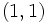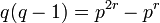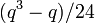# Subgroup structure of special linear group:SL(2,3)

View subgroup structure of particular groups | View other specific information about special linear group:SL(2,3)

This article gives information on the subgroup structure of special linear group:SL(2,3), which is the special linear group of degree two over field:F3. Note that this field has three elements$0,1,2$ and we have$2 = -1$.

## Tables for quick information

FACTS TO CHECK AGAINST FOR SUBGROUP STRUCTURE: (finite solvable group)
Lagrange's theorem (order of subgroup times index of subgroup equals order of whole group, so both divide it), |order of quotient group divides order of group (and equals index of corresponding normal subgroup)
Sylow subgroups exist, Sylow implies order-dominating, congruence condition on Sylow numbers|congruence condition on number of subgroups of given prime power order
Hall subgroups exist in finite solvable|Hall implies order-dominating in finite solvable| normal Hall implies permutably complemented, Hall retract implies order-conjugate
MINIMAL, MAXIMAL: minimal normal implies elementary abelian in finite solvable | maximal subgroup has prime power index in finite solvable group

### Quick summary

Item Value
number of subgroups 15
number of conjugacy classes of subgroups 7
number of automorphism classes of subgroups 7
isomorphism classes of Sylow subgroups, corresponding fusion systems, and Sylow numbers 2-Sylow: quaternion group (order 8) as Q8 in SL(2,3) with its non-inner fusion system (see non-inner fusion system for quaternion group), Sylow number 1
3-Sylow: cyclic group:Z3 with its non-inner fusion system, Sylow number 4
Hall subgroups The order has only two prime divisors, so no possibility of Hall subgroups other than trivial subgroup, whole group, and Sylow subgroups
maximal subgroups There are maximal subgroups of orders 6 (Z6 in SL(2,3)) and 8 (2-Sylow subgroup of special linear group:SL(2,3))
normal subgroups There are two proper nontrivial normal subgroups: center of special linear group:SL(2,3) and 2-Sylow subgroup of special linear group:SL(2,3)

### Table classifying subgroups up to automorphism

Note that, in the matrices, -1 can be written as 2 since elements are taken modulo 3.

Automorphism class of subgroups Representative subgroup Isomorphism class Order of subgroups Index of subgroups Number of conjugacy classes (=1 iff automorph-conjugate subgroup) Size of each conjugacy class (=1 iff normal subgroup) Total number of subgroups (=1 iff characteristic subgroup) Isomorphism class of quotient (if exists) Subnormal depth (if subnormal) Note
trivial subgroup$\{ \begin{pmatrix} 1 & 0 \\ 0 & 1 \\\end{pmatrix} \}$ trivial group 1 24 1 1 1 special linear group:SL(2,3) 1 trivial
center of special linear group:SL(2,3)$\{ \begin{pmatrix} 1 & 0 \\ 0 & 1 \\\end{pmatrix}, \begin{pmatrix} -1 & 0 \\ 0 & -1 \\\end{pmatrix} \}$ cyclic group:Z2 2 12 1 1 1 alternating group:A4 1
Z4 in SL(2,3)$\langle \begin{pmatrix} 0 & 1 \\ -1 & 0 \\\end{pmatrix}\rangle$ cyclic group:Z4 4 6 1 3 3 -- 2
2-Sylow subgroup of special linear group:SL(2,3)$\langle \begin{pmatrix} 0 & 1 \\ -1 & 0 \\\end{pmatrix}, \begin{pmatrix} -1 & -1 \\ -1 & 1 \\\end{pmatrix} \rangle$ quaternion group 8 3 1 1 1 cyclic group:Z3 1 2-Sylow
Z3 in SL(2,3)$\langle \begin{pmatrix} 1 & 1 \\ 0 & 1 \\\end{pmatrix} \rangle$ cyclic group:Z3 3 8 1 4 4 -- -- 3-Sylow
Z6 in SL(2,3)$\langle \begin{pmatrix} 1 & 1 \\ 0 & 1 \\\end{pmatrix}, \begin{pmatrix} -1 & 0 \\ 0 & -1 \\\end{pmatrix} \rangle$ cyclic group:Z6 6 4 1 4 4 -- -- 3-Sylow normalizer
whole group all elements special linear group:SL(2,3) 24 1 1 1 1 trivial group 0
Total (7 rows) -- -- -- -- 7 -- 15 -- -- --

### Table classifying isomorphism types of subgroups

Group name GAP ID Occurrences as subgroup Conjugacy classes of occurrence as subgroup Automorphism classes of occurrence as subgroup Occurrences as normal subgroup Occurrences as characteristic subgroup
Trivial group$(1,1)$ 1 1 1 1 1
Cyclic group:Z2$(2,1)$ 1 1 1 1 1
Cyclic group:Z3$(3,1)$ 4 1 1 0 0
Cyclic group:Z4$(4,1)$ 3 1 1 0 0
Cyclic group:Z6$(6,2)$ 4 1 1 0 0
Quaternion group$(8,4)$ 1 1 1 1 1
Special linear group:SL(2,3)$(24,3)$ 1 1 1 1 1
Total -- 15 7 7 4 4

### Table listing number of subgroups by order

Group order Occurrences as subgroup Conjugacy classes of occurrence as subgroup Occurrences as normal subgroup Occurrences as characteristic subgroup
1 1 1 1 1
2 1 1 1 1
3 4 1 0 0
4 3 1 0 0
6 4 1 0 0
8 1 1 1 1
24 1 1 1 1
Total 15 7 4 4

## Defining functions

### Subgroup-defining functions and associated quotient-defining functions

Subgroup-defining function What it means Value as subgroup Value as group Order Associated quotient-defining function Value as group Order (= index of subgroup)
center elements that commute with every group element center of special linear group:SL(2,3) cyclic group:Z2 2 inner automorphism group alternating group:A4 12
derived subgroup subgroup generated by all commutators 2-Sylow subgroup of special linear group:SL(2,3) quaternion group 8 abelianization cyclic group:Z3 3
second derived subgroup derived subgroup of derived subgroup center of special linear group:SL(2,3) cyclic group:Z2 2  ? alternating group:A4 12
perfect core the subgroup at which the derived series stabilizes trivial subgroup trivial group 1  ? special linear group:SL(2,3) 24
hypocenter the subgroup at which the lower central series stabilizes 2-Sylow subgroup of special linear group:SL(2,3) quaternion group 8  ? cyclic group:Z3 3
hypercenter the subgroup at which the upper central series stabilizes center of special linear group:SL(2,3) cyclic group:Z2 2  ? alternating group:A4 12
Frattini subgroup intersection of all maximal subgroups center of special linear group:SL(2,3) cyclic group:Z2 2 Frattini quotient alternating group:A4 12
Jacobson radical intersection of all maximal normal subgroups 2-Sylow subgroup of special linear group:SL(2,3) quaternion group 8  ? cyclic group:Z3 1
socle join of all minimal normal subgroups center of special linear group:SL(2,3) cyclic group:Z2 2 socle quotient alternating group:A4 12
Baer norm intersection of normalizers of all subgroups center of special linear group:SL(2,3) cyclic group:Z2 2  ? alternating group:A4 12
join of all abelian normal subgroups subgroup generated by all the abelian normal subgroups center of special linear group:SL(2,3) cyclic group:Z2 2  ? alternating group:A4 12
Fitting subgroup subgroup generated by all the nilpotent normal subgroups 2-Sylow subgroup of special linear group:SL(2,3) quaternion group 8  ? cyclic group:Z3 1
solvable radical subgroup generated by all the solvable normal subgroups whole group special linear group:SL(2,3) 24  ? trivial group 1

## Sylow subgroups

Compare and contrast with subgroup structure of special linear group of degree two over a finite field#Sylow subgroups

We are considering the group$SL(2,q)$ with$q = p^r$ a prime power,$q = 3, p = 3, r = 1$. The prime$p = 3$ is the characteristic prime.

### Sylow subgroups for the prime 3

The prime 3 is the characteristic prime$p$, so we compare with the general information on$p$-Sylow subgroups of$SL(2,q)$.

Item Value for$SL(2,q)$, generic$q$ Value for$SL(2,3)$ (so$q = p = 3, r = 1$)
order of$p$-Sylow subgroup$q$ 3
index of$p$-Sylow subgroup$q^2 - 1$ 8
explicit description of one of the$p$-Sylow subgroups unitriangular matrix group of degree two:$\{ \begin{pmatrix} 1 & b \\ 0 & 1 \\\end{pmatrix} \mid b \in \mathbb{F}_q \}$$\{ \begin{pmatrix} 1 & 0 \\ 0 & 1 \\\end{pmatrix},\begin{pmatrix} 1 & 1 \\ 0 & 1 \\\end{pmatrix},\begin{pmatrix} 1 & 2 \\ 0 & 1 \\\end{pmatrix}\}$. See 3-Sylow subgroup of special linear group:SL(2,3)
isomorphism class of$p$-Sylow subgroup additive group of the field$\mathbb{F}_q$, which is an elementary abelian group of order$p^r$ cyclic group:Z3
explicit description of$p$-Sylow normalizer Borel subgroup of degree two:$\{ \begin{pmatrix} a & b \\ 0 & a^{-1} \\\end{pmatrix} \mid a \in \mathbb{F}_q^\ast, b \in \mathbb{F}_q \}$ See Z6 in SL(2,3)
isomorphism class of$p$-Sylow normalizer It is the external semidirect product of$\mathbb{F}_q$ by the multiplicative group of$\mathbb{F}_q^\ast$ where the latter acts on the former via the multiplication action of the square of the acting element.
For$p = 2$ (so$q = 2,4,8,\dots$), it is isomorphic to the general affine group of degree one$GA(1,q)$.
For$q = 3$, it is cyclic group:Z6 and for$q = 5$, it is dicyclic group:Dic20.
cyclic group:Z6
order of$p$-Sylow normalizer$q(q - 1) = p^{2r} - p^r$ 6$p$-Sylow number (i.e., number of$p$-Sylow subgroups) = index of$p$-Sylow normalizer$q + 1$ (congruent to 1 mod p, as expected from the congruence condition on Sylow numbers) 4

### Sylow subgroups for the prime 2

We are in the subcase where$\ell = 2$ ($\ell$ being the prime for which we are taking Sylow subgroups) and$q \equiv 3 \pmod 8$.

Item Value for$\ell = 2, q \equiv 3 \pmod 8$ Value for$\ell = 2, q = p = 3, r = 1$ (our case)
order of 2-Sylow subgroup 8 8
index of 2-Sylow subgroup$(q^3 - q)/8$ 3
explicit description of one of the 2-Sylow subgroups PLACEHOLDER FOR INFORMATION TO BE FILLED IN: [SHOW MORE] See 2-Sylow subgroup of special linear group:SL(2,3)
isomorphism class of 2-Sylow subgroup quaternion group quaternion group
explicit description of 2-Sylow normalizer PLACEHOLDER FOR INFORMATION TO BE FILLED IN: [SHOW MORE] whole group
isomorphism class of 2-Sylow normalizer special linear group:SL(2,3) special linear group:SL(2,3)
order of 2-Sylow normalizer 24 24
2-Sylow number (i.e., number of 2-Sylow subgroups) = index of 2-Sylow normalizer$(q^3 - q)/24$ 1